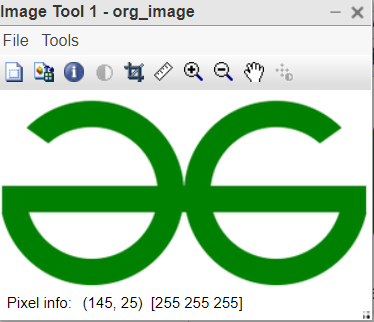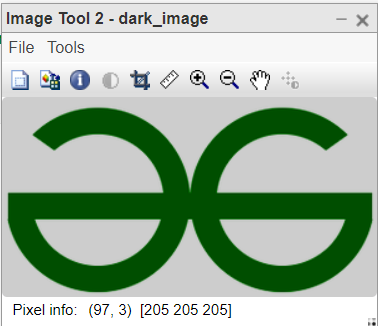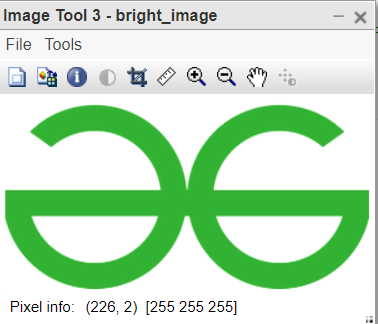GeeksforGeeks App
Open AppBrowser
Continue

# Increase and decrease the brightness of an image in MATLAB

The degree of the illuminance of the image is called brightness. How much light is coming out of an object defines the brightness of that object. If an object is placed in a dark room then very less light will be emitted by that object thus we can’t see the object properly. Now, what do we mean by brightness in the context of a digital image? It is defined as the intensity level of the pixels of the image. We know, 0 intensity means dark pixel and 1 intensity means white pixel. Therefore, brightness can be said as the average intensity value of all the pixels of an image.

Syntax:

image = image – C; where C is constant, like 50.

image = image + C; where C is constant, like 50.

imtool(image_var, [ ]);

Example 1:

## Matlab

 `% MATLAB code for read the image ``% and convert into dark and bright images.``org_image=imread(``"lady_with_hat.jfif"``);``dark_image=uint8(org_image-50);``bright_image=uint8(org_image+50);`` ` `% Bring the all three images to see``% the difference in brightness.``imtool(org_image, []);``imtool(dark_image, []);``imtool(bright_image, []);`

Output:Example 2:

## Matlab

 `% MATLAB code for read the colored image``% and convert into dark and bright images.``org_image=imread(``"logo.png"``); ``dark_image=uint8(org_image-50);``bright_image=uint8(org_image+50);`` ` `% Bring the all three images to see ``% the difference in brightness.``imtool(org_image, []);``imtool(dark_image, []);``imtool(bright_image, []);`

Output:To increase the brightness we have to increase the intensity of each pixel. Image is a collection of pixel values. So, they are additive in nature. We can just add a constant to every pixel value. To decrease the brightness we can just decrease a constant value from all the pixels of the image.

My Personal Notes arrow_drop_up